# Photochemistry & Beer-Lambert's Law Notes | Study Physical Chemistry - Chemistry

## Chemistry: Photochemistry & Beer-Lambert's Law Notes | Study Physical Chemistry - Chemistry

The document Photochemistry & Beer-Lambert's Law Notes | Study Physical Chemistry - Chemistry is a part of the Chemistry Course Physical Chemistry.
All you need of Chemistry at this link: Chemistry

Photochemistry

Photochemistry process involves the initiation of a chemical reaction through the absorption of a photon by an atom or molecule.

• When a molecule absorbs a photon of light, the energy of photon is transferred to the molecule. The energy of a photon is given by the Planck equation: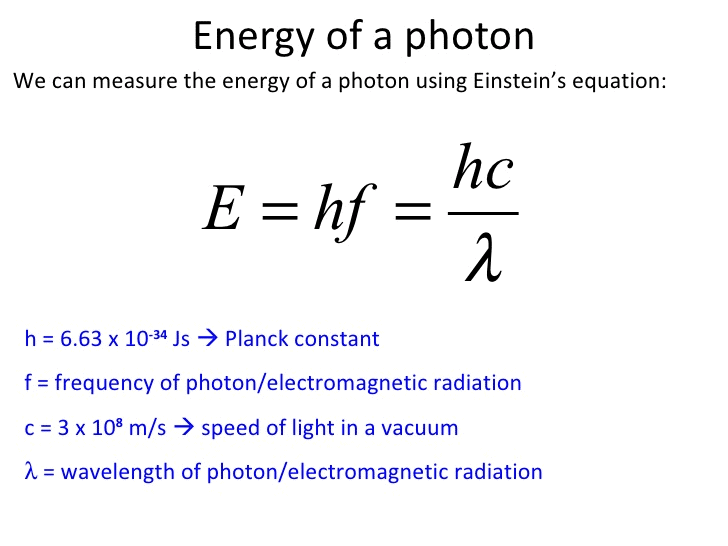h = Planck constant = 6.626 × 10–34 J-s
c = speed of light in vacuum = 3 × 108 ms–1
v = frequency of light
and λ = wavelength of light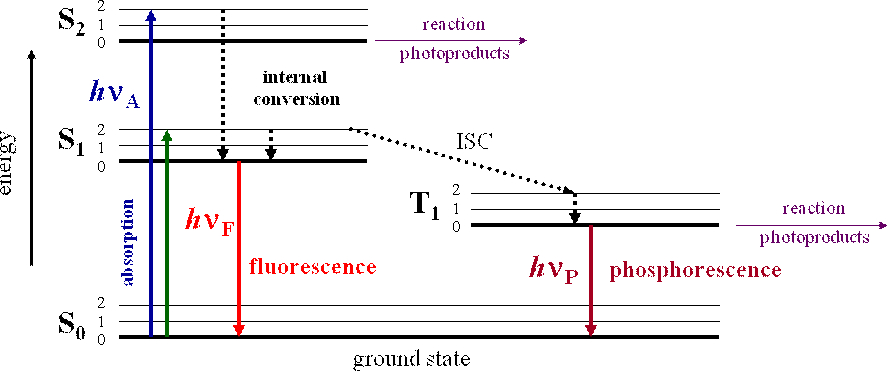So, S1, S2, T1 & T2 are electronic levels.
S → singlet
T → triplet

• Radiative Transition: Loss of excess electronic energy through the emission of a photon is known as radiative transition.
•  Fluorescence: The process by which photons are emitted in radiative transit io n between S1 and S0 is known as fluorescence.
• Phosphorescence: The process by which photons are emitted in radiative transit io n between T1 and S0 is known as phosphorescence.
• The lifetime for phosphorescence is longer (10–6 s) than fluorescence (10–9 s) Photophysical reactions are corresponding rate expressions.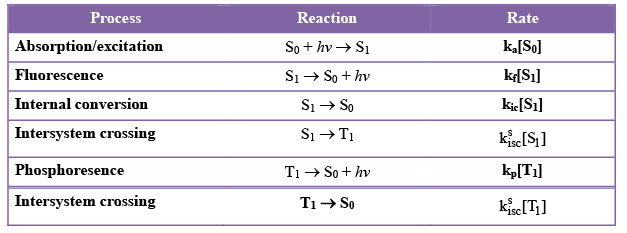• Quantum Yield: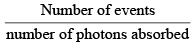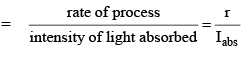The Beer-Lambert Law.

The Beer-Lambert law states that for a given material sample path length and concentration of the sample are directly proportional to the absorbance of the light.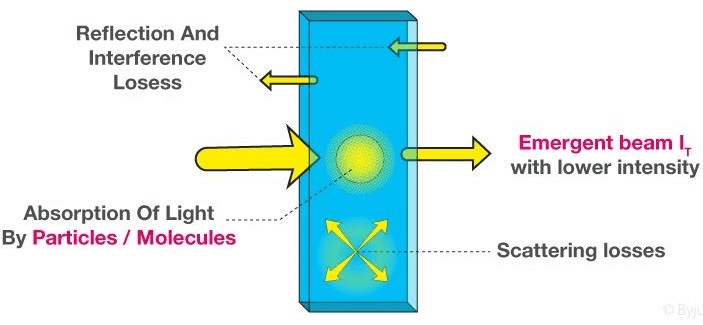• Beer-Lambert Law derivation helps us to define the relationship of the intensity of visible UV radiation with the exact quantity of substance present.
• The derivation of Beer-Lambert Law has many applications in modern-day science. Used in modern-day labs for testing of medicines, organic chemistry, and to test with quantification. These are some fields that this law finds its uses in.
• When a beam of monochromatic radiation of a suitable frequency passes through a solution, it is absorbed by the solution.

The Beer-Lambert law is expressed as:

A = εLc

where,

• A is the amount of light absorbed for a particular wavelength by the sample
• ε is the molar extinction coefficient
• L is the distance covered by the light through the solution
• c is the concentration of the absorbing species

But Beer-Lambert law is a combination of two different laws: Beer’s law and Lambert law.

Derivation of Absorbance and Transmittance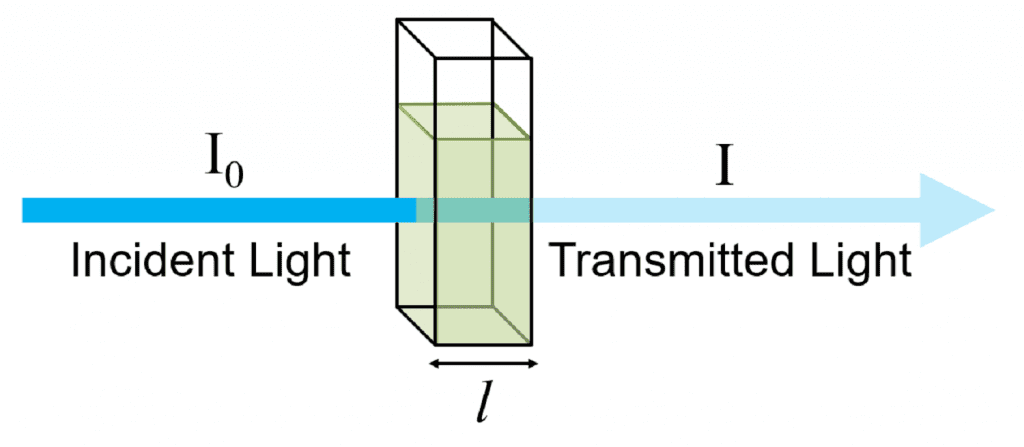I0 = intensit y of incident light
I = intensity of transmitted light
and Ia = Intensity of the light absorbed = I0 – I
Absorbance of solution, A =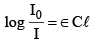A =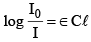…(1)

where A = absorbance
C = concentration of solution
∈ = molar extinction coefficient or molar absorption coefficient (unit = concentration length–1)
l = path length

Transmittance;

T =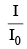T =…(2)

The absorbance of a solution is additive whereas the transmittance is multiplicative.

Some Important Questions

Q.1. A monochromatic light is an incident on the solution of 0.05 molar concentration of an absorbing substance. The intensity of the radiation is reduced to one-fourth of the initial value after passing through 10 cm length of the solution. Calculate the molar extinction coefficient of the substance.
Sol.
From bear Lambert law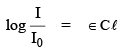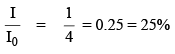i.e.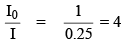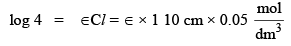2 × 0.3010 = 0.5 ∈
∈ = 1.204 dm3 mol–1 cm–1

Q.2. A substance when dissolved in water at 10–3 M concentration absorbs 10 percent of incident radiation in a path of 1 cm length.  What should be the concentration of the solution in order to absorb go percent of the same solution?
Sol.
∵ 10% absorbed then 90% transmitted
then     T =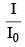= 90%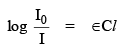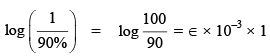…(1)

∵ 90% absorbed then 10% transmitted
then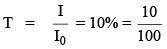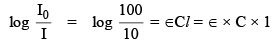…(2)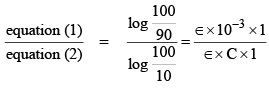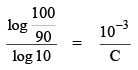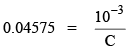C = 0.0218 mol dm–3.

Q.3. In carbon-dating application of radioisotopes, 14C emits
(1) β-particle (2) α-particle (3) γ--particle (4) positron

Sol.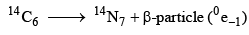Q.4. With the increase in temperature, Gibb’s free energy for the adsorption of a gas on a solid surface.
(1) Becomes more positive from a positive value
(2) Becomes more negative from a positive
(3) Becomes more positive from a negative value
(4) Becomes more negative from a negative value

Sol.  From Langmuir isotherm, the fractional coverage is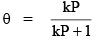∵ P ∝ T
Then higher the pressure or temperature lower the value of fractional coverage q.
R(g) + M(surface)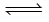RM (surface)

if q decreases then the formation of RM decreases. i.e. rate of formation of RM decreases.
This indicates that Gibbs from the energy of adsorption becomes positive.
So, increase the temperature, the Gibbs free energy of adsorption of a gas on a solid surface become more positive from a negative value.

Q.5. One of the assumptions made in the conventional activated complex theory is:
(1) Equilibrium is maintained between the reactants and the activated
(2) Equilibrium is maintained between the reactants and the product
(3) Equilibrium is maintained between the products and the activated complex
(4) Equilibrium is maintained between the reactants, the activated complex and the products
Sol.
According to Eyring, the equilibrium is maintained between reactants and the activated complex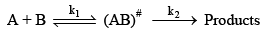(AB)# is the activated complex.

Q.6. For a reaction, the rate constant k at 27°C was found to be k = 5.4 × 1011 e–50 The activation energy of the reaction is
(1) 50 J mol–1 (2) 415 J mol–1 (3) 15000 J mol–1 (4) 125000 J mol–1

Sol.  From Arrhenius equation

k = Ae-Ea / RT &(1)
&
k = 5.4 × 1011 e–50 …(2)

From equation (1) & (2) we get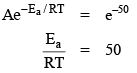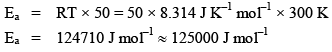The document Photochemistry & Beer-Lambert's Law Notes | Study Physical Chemistry - Chemistry is a part of the Chemistry Course Physical Chemistry.
All you need of Chemistry at this link: ChemistryUse Code STAYHOME200 and get INR 200 additional OFF

## Physical Chemistry

83 videos|106 docs|31 tests

Track your progress, build streaks, highlight & save important lessons and more!

,

,

,

,

,

,

,

,

,

,

,

,

,

,

,

,

,

,

,

,

,

;